Courses

# Modern Physics MCQ Level - 1

## 45 Questions MCQ Test | Modern Physics MCQ Level - 1

Description
This mock test of Modern Physics MCQ Level - 1 for Class 12 helps you for every Class 12 entrance exam. This contains 45 Multiple Choice Questions for Class 12 Modern Physics MCQ Level - 1 (mcq) to study with solutions a complete question bank. The solved questions answers in this Modern Physics MCQ Level - 1 quiz give you a good mix of easy questions and tough questions. Class 12 students definitely take this Modern Physics MCQ Level - 1 exercise for a better result in the exam. You can find other Modern Physics MCQ Level - 1 extra questions, long questions & short questions for Class 12 on EduRev as well by searching above.
QUESTION: 1

Solution:
QUESTION: 2

Solution:
QUESTION: 3

### In a α -decay, the kinetic energy of a-particle is 48 MeVand Q value of the reaction is 50 MeV. The mass numberof the mother nucleus is (assume that daughter nucleusis in ground state)

Solution:
QUESTION: 4

A sample of radioactive material decays simultaneouslyby with two processes A and B with half lives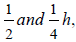,respectively. For first half hour it decays with the processA. next one hour with the process B, and for further halfan hour with both A and B. If originally there were N0nuclei, find the number of nuclei after 2 h of such decay.

Solution:
QUESTION: 5

In which of the following processes, the number of protonsthe nucleus increase?

Solution:
QUESTION: 6

A radioactive nucleus ‘X’ decays to a stable nucleus ‘Y’. Then, time graph of rate of formation of ‘Y’ against time 't' will be:

Solution:
QUESTION: 7

A heavy nucleus having mass number 200 getsdisintegrated ‘into two small fragments of mass numbers80 and 120. If binding energy per nucleon for parent atomis 6.5 MeV and for daughter nuclei is 7 MeV and 8 MeV,respectively, then the energy released in the decay will be

Solution:
QUESTION: 8

An element X decays, first by positron emission and thentwo a -particles are emitted in successive radioactivedecay. If the product nucleus has a mass number 229and atomic number 89, the mass number and atomicnumber of element Xare

Solution:
QUESTION: 9

90% of a radioactive sample is left undecayed aftertime t has elapsed. What percentage of the initialsample will decay in a total time 2t?

Solution:
QUESTION: 10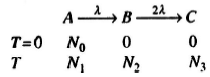The ratio of /V, to N2 when ,V2 is maximum is

Solution:
QUESTION: 11

The binding energy of an electron in the ground stateof he atom is E0 = 24.6 eV. The energy required toremove both the electrons from the atom is

Solution:
QUESTION: 12

The mean life time of a radionuclide, if its activitydecreases by 4% for every 1 h. would be (product isnon-radioactive, i.e., stable)

Solution:
QUESTION: 13

On an average, a neutron loses half of its energy percollision with a quasi-free proton. To reduce a 2 MeVneutron to a thermal neutron having eneigy 0.04 eV.the number of collisions required is nearly

Solution:
QUESTION: 14

Atomic masses of two isobars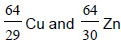are 63.9298 and 63.9292 u, respectively. It can beconcluded from this data that

Solution:
QUESTION: 15

If a nucleus such as 226Ra that is initially at restundergoes alpha decay, then which of the followingstatements is true?

Solution:
QUESTION: 16

1.00 kg of 235U undergoes fission process. If energy released per event is 200 MeV, then the total energy released

Solution:
QUESTION: 17

Mark out the incorrect statement.

Solution:
QUESTION: 18

U-235 can decay by many ways, let us here consideronly v_^iwo ways A and B. In decay of U-235 by meansof A. the energy released per fission is 210 MeV whilein B it is 186 MeV. Then, the uranium 235 sample ismore likely to decay by

Solution:
QUESTION: 19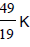isotope of potassium has a half-life of 1.4 x 10yr and decays to form stable argon,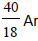. A sample of rock has been taken which contains both potassium and argon in the ratio 1:7, i.e.,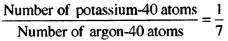Assuming that when the rock was formed no argon-40 was present in the sample and none has escaped subsequently, determine the age of the rock,

Solution:
QUESTION: 20

The probability of survival of a radioactive nucleus forone mean life is

Solution:
QUESTION: 21

Two radioactive materials X1 and X2 have decayconstants10λ and λ , respectively. If initially they havethe same number of nuclei, the ratio of the number ofnuclei of X1 to that of X2 will be 1/e after a time

Solution:
QUESTION: 22

A radioactive substance is being consumed at aconstant rate of 1 s-1. After what time will the number of radioactive nuclei become 100. Initially, there were 200 nuclei present.

Solution:
QUESTION: 23

A radioactive substance X decays into anotherradioactive substance Y. Initially, only X was present.λx and λy Ay are the disintegration constants of X andY. Ny will be maximum when

Solution:
QUESTION: 24

There are two radio nuclei A and B. A is an alpha emitterand B a beta emitter. Their disintegration constantsare in the ratio of 1:2. What should be the ratio of numberof atoms of A and B at any time t so that probabilitiesof getting alpha and beta particles are same at thatinstant?

Solution:
QUESTION: 25

Half-life of a radioactive substance A is two times thehalf- life of another radioactive substance B. Initially,the number of A and B are NA and NB, respectively.After three half- lives of A, number of nuclei of both areequal. Then, the ratio NA/NB is

Solution:
QUESTION: 26

A radioactive nucleus is being produced at a constantrate a per second. Its decay constant is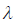. If N0 arethe number of nuclei at time t = 0, then maximumnumber of nuclei possible are

Solution:
QUESTION: 27

In a sample of a radioactive substance, what fractionof the initial nuclei will remain undecayed after a timet = T/2, where T = half-life of radioactive substance?

Solution:
QUESTION: 28

The activity of a radioactive substance is R1 at time t1and R2 at time t2 (> t1). Its decay constant is. Then

Solution:
QUESTION: 29

The ratio of molecular mass of two radioactivesubstances is 3/2 and the ratio of their decay constantis4/3 , Then, the ratio of their initial activity per mole will be

Solution:
QUESTION: 30

N1 atoms of a radioactive element emit N2 beta particlesper second. The decay constant of the element is (in s-1)

Solution:
QUESTION: 31

The binding energies of nuclei X and Y are E1 and E2,respectively. two atoms of X fuse to give one atom of Yand an energy Q is released. Then,

Solution:
QUESTION: 32

The activity of a radioactive element decreases to onethirdof the original activity A0 in a period of 9 years.After a further lapse of 9 years, its activity will be

Solution:
QUESTION: 33

The activity of a radioactive sample is 1.6 curie, and itshalf life is 2.5 days. Its activity after 10 days will be

Solution:
QUESTION: 34

The half-life of a certain radioactive isotope is 32 h.What fraction of a sample would remain after 16 h?

Solution:
QUESTION: 35

Samples of two radioactive nuclides, X and Y, eachhave equal activity A at time t=0. X has a half-life of 24years and Y a half-life of 16 years. The samples aremixed together. What will be the total activity of themixture at t=48 years?

Solution:
QUESTION: 36

Atomic mass number of an element is 232 and its atomic number is 90. The end product of this radioactive element is an isotope of lead (atomic mass 208 and atomic number 82). The number of α and β -particles emitted are

Solution:
QUESTION: 37

A radioactive nucleus undergoes a series of decaysAccording to the schemeIf the mass number and atomic number of A are 180and 72, respectively, then what are these number forA4?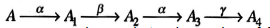Solution:
QUESTION: 38

The activity of a radioactive element decreases to onethirdof the original activity /0 in a period of nine years.After a further lapse of nine years, its activity will be

Solution:
QUESTION: 39

The half-life period of RaB(82Pb214) is 26.8 min. The massof one curie of RaB is

Solution:
QUESTION: 40

Half lives of two radioactive substances A and B are,respectively, 20 min and 40 min. Initially, the samplesof A and B have equal number of nuclei. After 80 min.the ratio of the remaining number of A and B nuclei is

Solution:
QUESTION: 41

A radioactive nucleus can decay by two different processes. The mean value period for the first processis t1 and that for the second process is t2. The effectivemean value period for the two processes is

Solution:
QUESTION: 42

The half-life of radium is 1620 years and its atomicweight is 226. The number of atoms that will decayfrom its 1 g sample per second will be

Solution:
QUESTION: 43

Assuming that about 200 MeV of energy is releasedper fission of 92U235 nuclei, the mass of U235 consumedper day in a fission reactor of power 1 megawatt will beapproximately

Solution:
QUESTION: 44

A radio isotope 'X' has a half-life of 10 s. Find the numberof nuclei in the sample (if initially there are 1000isotopes which are falling from rest from a height of3000 m) when it is at a height of 1000 m from the reference plane

Solution:
QUESTION: 45

In the nuclear reaction given by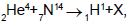thenucleus X is

Solution: